#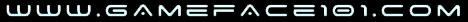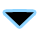EVENTALIST
EVENTALIST
#1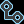Simple Colors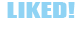#### mr_wiggles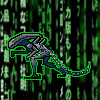EVENTALIST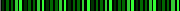EVENTALIST
profile## SimpleColor

• Author: Mr_Wiggles | Version: 1.0 | Date: 11/19/15

#### Description:

This was a script I decided to put together because i didn't like how you had to look up the color every time you call it in script. Browsing all the way to the Img directory and then system and count tiles... time consuming.
Of course you always have:

var color = 'rgba(255,255,255,255)'

But that takes an art and patience to fine tune in even the basic colors on the wheel. This script will allow you to use a simple command to get at least a simple color shade off the color wheel by using a standard string.

#### Screen Shots:

Colors Currently in the list:#### Version History:

• Version 1.0 (11/19/15) - Initial release.

#### Script:

Code:
`//================================================================================// SimpleColor.js//================================================================================/*: @author Mr_Wiggles | Date: 11/19/15 | Version: 1.0 * ------------------------------------------------------------------------------- * @plugindesc Makes it easier to use colors when scripting. * * @help This plugin is more for scripters then it is for any other part involving * RMMV. It simplfies the color codes to that of string commands.  * * Information on the built in Bitmap class. * http://developer.android.com/reference/android/graphics/Bitmap.html * * Information about the built in Array class: * https://developer.mozilla.org/en-US/docs/Web/JavaScript/Reference/ *   Global_Objects/Array/includes * ------------------------------------------------------------------------------ * To use in a javascript file: *      var color = \$gameTemp.getColor('blue'); *      var color = \$gameTemp.getColor('VL green'); *      var color = \$gameTemp.getColor('D orange'); *      var color = \$gameTemp.getColor('L grey'); *      var color = \$gameTemp.getColor('VD purple'); * *    All the base colors are included; The primary [red, yellow, blue] and the * secondary colors [green, orange, purple]. Along with black, white, and  * grey/gray of course. You also have extra tags before the color name like 'D'  * for dark 'L" for light and you can add 'V' infront of those two for a 'very'  *                     tag, so 'VD' very dark. *             -------------------------------------------- *        You might also want to use a conditional branch like  *                    the one illustrated bellow: * * if (PluginManager._scripts.contains('SimpleColor')) {// SimpleColor is in list. *   try { var color = \$gameTemp.sampleAllColors(); *   } catch(e) {throw new Error('SimpleColor failed to load. ' + e); } * } else { // Simple color isn't being used, just go defualt method. *   var color = Window_Object.textColor(0); * } * * The above fail safe if statement will make sure there is still an option to * set the color variable if this script is not loaded into the uers plugin list * along with your plugin script. * ------------------------------------------------------------------------------ * If you'd like there is a way to view all the colors layed out on one bitmap * with their respective names you can use: *   var sample = \$gameTemp.sampleAllColors(); *   this.bitmap.blt(sample, 0, 0, sample.width, sample.height, 0, 0); * * It will also display the names used to retrive said color as well. Currently * "\$gameTemp.sampleAllColors()" returns a bitmap that is 320 by 96 pixels. * ------------------------------------------------------------------------------ * Can't make your mind up on a color? Try using \$gameTemp.getRandomColor() *//* ---------------------------------------------------------------------------- */ //============================================================================(function() {//--------------------------------------------------------------------------------// Pulls color data using string name.  Game_Temp.prototype.getColor = function(string, opacity) {    var o = opacity || 255;    var r = 0; var g = 0; var b = 0;    if (string.contains('red')) {         // RED  --------------------------------      r = 205; g = 0; b = 20;      if (string.contains('V')) {        if (string.contains('L')) {r = 255; g = 120; b = 125; } else if (string.contains('D')) {r = 120; g = 0; b = 0; }      } else {        if (string.contains('L')) {r = 250; g = 95; b = 95; } else if (string.contains('D')) {r = 155; g = 0; b = 0; }      }    } else if (string.contains('blue')) { // BLUE --------------------------------      r = 20; g = 0; b = 195;      if (string.contains('V')) {        if (string.contains('L')) {r = 0; g = 150; b = 255; } else if (string.contains('D')) {r = 30; g = 0; b = 120; }      } else {        if (string.contains('L')) {r = 30; g = 90; b = 255; } else if (string.contains('D')) {r = 0; g = 45; b = 165; }      }    } else if (string.contains('yellow')) {// YELLOW -----------------------------      r = 230; g = 230; b = 0;      if (string.contains('V')) {        if (string.contains('L')) {r = 255; g = 255; b = 190; } else if (string.contains('D')) {r = 160; g = 160; b = 0; }      } else {        if (string.contains('L')) {r = 255; g = 255; b = 140; } else if (string.contains('D')) {r = 200; g = 200; b = 0; }      }    } else if (string.contains('green')) { // GREEN ------------------------------      r = 0; g = 200; b = 0;      if (string.contains('V')) {        if (string.contains('L')) {r = 150; g = 240; b = 150; } else if (string.contains('D')) {r = 0; g = 110; b = 0; }      } else {        if (string.contains('L')) {r = 50; g = 240; b = 50; } else if (string.contains('D')) {r = 0; g = 150; b = 0; }      }    } else if (string.contains('orange')) {// ORANGE -----------------------------      r = 245; g = 130; b = 50;      if (string.contains('V')) {        if (string.contains('L')) {r = 255; g = 190; b = 90; } else if (string.contains('D')) {r = 165; g = 90; b = 0; }      } else {        if (string.contains('L')) {r = 255; g = 150; b = 40; } else if (string.contains('D')) {r = 205; g = 100; b = 10; }      }    } else if (string.contains('purple')) {// PURPLE -----------------------------      r = 200; g = 45; b = 200;      if (string.contains('V')) {        if (string.contains('L')) {r = 255; g = 160; b = 255; } else if (string.contains('D')) {r = 130; g = 10; b = 130; }      } else {        if (string.contains('L')) {r = 250; g = 85; b = 250; } else if (string.contains('D')) {r = 170; g = 15; b = 170; }      }    } else if (string.contains('white')) { // WHITE ------------------------------      r = 255; g = 255; b = 255;    } else if (string.contains('grey') || // GRAY or GREY ------------------------                 string.contains('gray')) {      r = 165; g = 165; b = 165;      if (string.contains('V')) {        if (string.contains('L')) {r = 230; g = 230; b = 230; } else if (string.contains('D')) {r = 85; g = 85; b = 85; }      } else {        if (string.contains('L')) {r = 190; g = 190; b = 190; } else if (string.contains('D')) {r = 120; g = 120; b = 120; }      }    } else if (string.contains('black')) { // BLACK ------------------------------      r = 0; g = 0; b = 0;    }    return new String('rgba('+r+','+g+','+b+','+o+')');  };  //------------------------------------------------------------------------------  // Returns a bitmap with all the colors layed out in tiles for sampling.  Game_Temp.prototype.sampleAllColors = function() {    var allColors = ['red', 'blue', 'orange', 'green', 'yellow', 'purple'];    var tileSize = 32;    var width = (allColors.length / 3) * 5 * tileSize;    var height = (tileSize * 2) + (Math.ceil(allColors.length / 3) * tileSize);    var rowMax = 10; var index = 0;    var bitmap = new Bitmap(width, height);    bitmap.fontSize = 8;    var x = 0; var y = 0; var color = null;    var colorString = ''; var extras = ['VL', 'L', '', 'D', 'VD'];    for (tile in allColors) {      var stringColor = allColors[tile];      if (typeof stringColor === 'string') {        for (extra in extras) {          var tag = extras[extra] // Arrays have header data that isn't needed ATM.          if (typeof tag === 'string') { // So needs to be sorted out and just use contents.            colorString = tag + ' ' + stringColor;            color = this.getColor(colorString);            x = (index % rowMax) * tileSize;            y = Math.floor(index / rowMax) * tileSize;            bitmap.fillRect(x, y, tileSize, tileSize, color);            bitmap.drawText(colorString, x + 2, y, tileSize - 4, tileSize, 'center');            index++;          }        } // end for tag      }    } // end for color    index = 0; y = y + tileSize;    color = this.getColor('white');    var offset = (tileSize * 2) + (tileSize / 2);    bitmap.fillRect(tileSize + (tileSize / 2), y, tileSize, tileSize, color);    bitmap.drawText('white', tileSize  + (tileSize / 2), y, tileSize - 4, tileSize, 'center');    for (extra in extras) {      var tag = extras[extra]      if (typeof tag === 'string') {         colorString = tag + ' gray';        color = this.getColor(colorString);        x = (index % rowMax) * tileSize;        bitmap.fillRect(x + offset, y, tileSize, tileSize, color);        bitmap.drawText(colorString, offset + x + 2, y, tileSize - 4, tileSize, 'center');        index++;      }    }    color = this.getColor('black');    bitmap.fillRect((tileSize * 7) + (tileSize / 2), y, tileSize, tileSize, color);    bitmap.drawText('black', (tileSize * 7) + (tileSize / 2), y, tileSize - 4, tileSize, 'center');    return bitmap;  };  //------------------------------------------------------------------------------  // Retrives a "random" color when ever called.  Game_Temp.prototype.getRandomColor = function() {    var r = Math.randomInt(255);    var g = Math.randomInt(255);    var b = Math.randomInt(255);    return 'rgba('+r+','+g+','+b+',255)';  };//================================================================================})(); // End of plugin block.`

#### Plugin Usage:

All you need to do is load the plugin into the GUI data base in RMMV, after you can follow the steps in the Help documentation for usage and such.

For the most part its as simple as:
Code:
`var iWantColor = \$gameTemp.getColor('L blue');`

And you have the color Light Blue saved to that variable and ready for direct use any where a color variable is required.

Even has a random feature:
Code:
`var iWantColor = \$gameTemp.getRandomColor();`
EVENTALIST
Show Signature
EVENTALIST